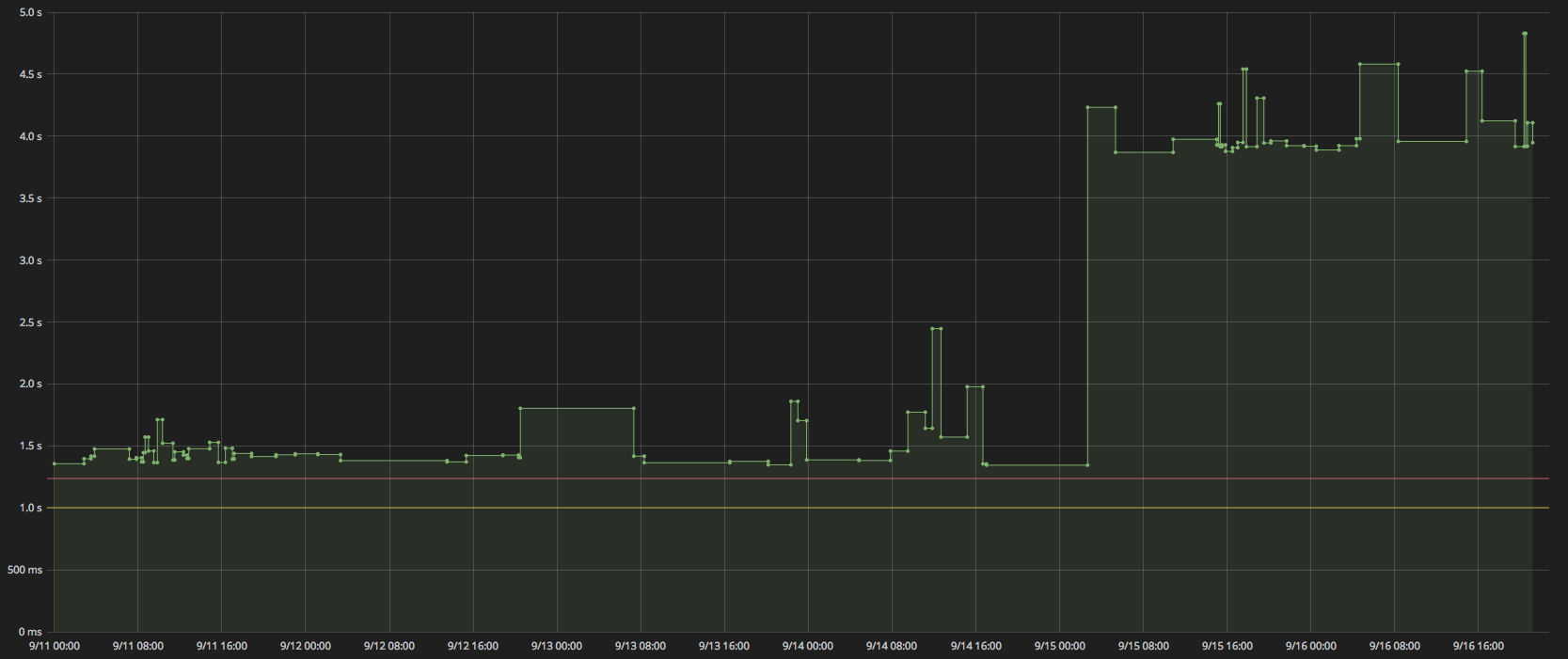# Determine breakpoints in time series data where values change significantly

Given the following chart of data:which represents duration metrics gathered at absolute times, I am trying to determine when/if any of the values exhibit a jump in durations. In the example chart, the trend of data appears to hover around the 1.4 second mark, while later in the chart, at a particular moment, the data hovers under 4 seconds.

Given a collection of data points similar to the one that produced this chart, is there a statistical function that can find the significant breakpoints in the data? I am a statistics n00b, but doing some research has led me into piecewise regression, but I do not understand it well enough to determine if it is the appropriate route.

For what it's worth, I'll be performing the analysis in JavaScript, so external libraries that have said function probably won't be of much use. I'm more looking for the actual math to arrive at the conclusion so that I may translate that into JavaScript.

• Eli, welcome! Great question. Lots of follow ups. Will this model be given "labeled" data that identifies when spikes happened? Is the goal to look at this data in real time and say after each tick, "Spike" or "No Spike"? Or will it be looking back after the fact and be able to utilize information to the right of the "spike" to determine if it was a spike? – jlimahaverford Sep 30 '15 at 20:12
• @jlimahaverford thanks! The goal is to query the data every day for the previous 7 days, then analyze that 7 days worth of data to see if a spike occurs in it, and if it does: where? Eventually I could annotate the existing data with where the spikes occurred, but I imagine that being trivial once I know where the proposed spikes are. :) (Please let me know if there are more follow-ups!) – Eli Sep 30 '15 at 20:19
• Also, let me know if I didn't actually answer your question. :P – Eli Sep 30 '15 at 20:20
• You kind of did. Is the following acceptable "Yesterday might have spiked, but I need to wait a day or two to know for sure?" Also, when I asked about labelled data, I meant would you be willing to look back at past skipes and identify them in order to help the training of the algorithm, or do you want the algorithm to identify them completely on their own. – jlimahaverford Sep 30 '15 at 20:22
• Yes, that is acceptable. I understand that you probably need to accumulate an amount of data before you can prove a trend change rather than just some temporary out of band variance. With regards to training, it's not necessary to my use case, but wouldn't be a problem if the formula needed it. If the formula identifies regressions previously found, that's not a problem for me. – Eli Sep 30 '15 at 20:39

Draw a graph of differences between observations. If your plot is $x_t$, then it would be $\Delta x_t=x_t-x_{t-1}$. You'll see that it'll have a big spike in the middle. If you calculate the standard deviation before and after the spike, you'd see that it's similar. You'll see that the magnitude of the spike is 2-3 times higher than of these standard deviations. This gives you an idea for modeling the series.

Start with a simple linear and symmetric model: $$\Delta x_t = \varepsilon_t,$$ where $\varepsilon\sim\mathcal{N}(0,\sigma^2)$ the Normal Distribution with mean 0 and variance $\sigma^2$.

This is how it works. Calculate the differences $\Delta x_t$ for each observation period. Remove the outliers either manually or using something like Grubbs test. The outliers are the big jumps.

Once outliers are removed, calculate the standard deviation of differences $\sigma=\sqrt{Var[\Delta x_t]}$. You can do this in Excel, for example.

Now, going forward, keep computing $\Delta x_t$ for future periods, whenever it's large, e.g. $\Delta x_t>2\sigma$ you'll say that there's 95% probability that it's a big jump. You can go for $3\sigma$, then the probability is 99.5%.

The problem with this approach is that if you draw a histogram of $\Delta x_t$, most likely it'll be skewed. So, Gaussian distribution will not be a good fit, but what I described could be a starting point for your analysis. You need to study the distribution of differences and spikes to find a good fit.

• The first paragraph kinda made sense, but I don't understand anything about the second, sorry. – Eli Sep 30 '15 at 20:40
• Could you maybe elaborate on what the different symbols stand for and which of my terms would map to these variables? – Eli Oct 1 '15 at 17:23

What you are looking for is change point detection. There is an extensive literature on this topic, and there are freely available software packages for performing this type of analysis. For example, see this paper on the R package changepoint which includes a brief survey of the literature, along with examples demonstrating how to perform this kind of analysis.